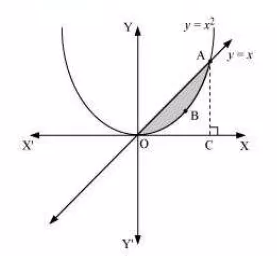Q

# Find the area between the curves y = x and y = x^2.

Q : 2         Find the area between the curves and .

Views

the area between the curves and .The curves intersect at A(1,1)
Draw a normal to AC to OC(x-axis)
therefore, the required area (OBAO)= area of (OCAO) - area of (OCABO)

Thus the area of shaded region is 1/6 units

Exams
Articles
Questions